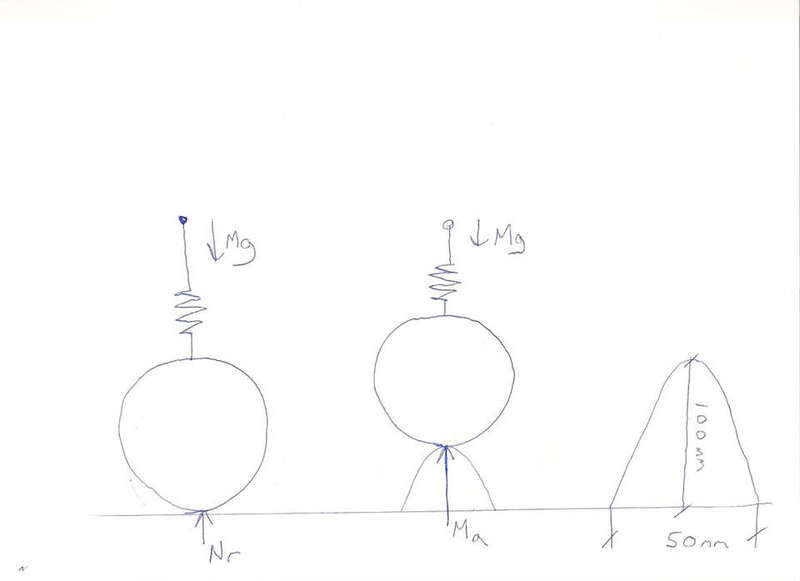# Force of wheel Perependicular to road over speed hump

Im curently trying to work out the upwards force of the wheel of a car as it passes over a hump in the road. I have drawn a very simple diagram to illustrate this. If anyone could help me calculate this force that would be most helpful.

I understand as the wheel is traveling along the road its normal reaction force (Nr) upwards is equal to that of the downwards force of the car (Mg) however when the wheel travels over the speed hump the normal reaction force increase as it is gains a greater upwards force (Ma).

if the car was traveling over the speed hump at 20 meteres per second what would be the upwards force of the wheel Ma ?tiny-tim
hi cjh1990!if the road is curved, then the vertical acceleration = … ?, so normal force = weight ± … ?